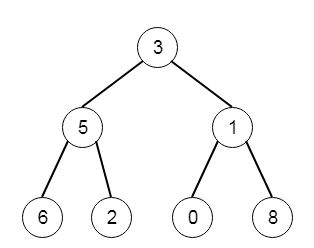# Find Largest Value in Each Tree Row in C++

C++Server Side ProgrammingProgramming

#### C in Depth: The Complete C Programming Guide for Beginners

45 Lectures 4.5 hours

#### Practical C++: Learn C++ Basics Step by Step

Most Popular

50 Lectures 4.5 hours

#### Master C and Embedded C Programming- Learn as you go

66 Lectures 5.5 hours

Suppose we have a binary tree, we have to find the largest elements of each level of that tree. So if the tree is like −Then the output will be [3,5,8]

To solve this, we will follow these steps −

• Define an array called ans

• define a recursive function solve(), this will take tree node, and level, the level is initially 0. this method will act like −

• if node is null, then return

• if level = size of ans, then insert node value into ans, otherwise ans[level] := max of ans[level] and node value

• call solve(left subtree of node, level + 1)

• call solve(right subtree of node, level + 1)

• From the main method, call the solve() using root as parameter, and level = 0

• Then return ans

Let us see the following implementation to get better understanding −

## Example

Live Demo

#include <bits/stdc++.h>
using namespace std;
void print_vector(vector<auto> v){
cout << "[";
for(int i = 0; i<v.size(); i++){
cout << v[i] << ", ";
}
cout << "]"<<endl;
}
class TreeNode{
public:
int val;
TreeNode *left, *right;
TreeNode(int data){
val = data;
left = NULL;
right = NULL;
}
};
void insert(TreeNode **root, int val){
queue<TreeNode*> q;
q.push(*root);
while(q.size()){
TreeNode *temp = q.front();
q.pop();
if(!temp->left){
if(val != NULL)
temp->left = new TreeNode(val);
else
temp->left = new TreeNode(0);
return;
} else {
q.push(temp->left);
}
if(!temp->right){
if(val != NULL)
temp->right = new TreeNode(val);
else
temp->right = new TreeNode(0);
return;
} else {
q.push(temp->right);
}
}
}
TreeNode *make_tree(vector<int> v){
TreeNode *root = new TreeNode(v);
for(int i = 1; i<v.size(); i++){
insert(&root, v[i]);
}
return root;
}
class Solution {
public:
vector <int> ans;
void solve(TreeNode* node, int level = 0){
if(!node)return;
if(level == ans.size()){
ans.push_back(node->val);
} else {
ans[level] = max(ans[level], node->val);
}
solve(node->left, level + 1);
solve(node->right, level + 1);
}
vector<int> largestValues(TreeNode* root) {
solve(root);
return ans;
}
};
main(){
vector<int> v = {1,3,2,5,3,NULL,9};
TreeNode *tree = make_tree(v);
Solution ob;
print_vector(ob.largestValues(tree));
}

## Input

[1,3,2,5,3,null,9]

## Output

[1, 3, 9, ]